• 简单理解RNN循环神经网络RNN(Recurrent Neural Network）介绍图说RNN宏观结构图说RNN微观结构 RNN(Recurrent Neural Network）介绍 介绍： RNN，也就是循环神经网络，是用来建模序列化数据的一种主流的深度学习模型...
• RNN循环神经网络实例

千次阅读 2019-04-22 21:56:20
最近又忍不住把RNN这一块儿的东西...关于RNN循环神经网络的具体概念我就不细讲了，稍微把概念给提下吧，然后会说说其变形模型，以及会给出两个demo讲述其在不同领域的应用，方便大家理解！ 1. RNN循环神经网络介绍 ...

最近又忍不住把RNN这一块儿的东西给过了一遍，感觉还是有一些收获！所以想着给记录下来，因为也看到有人给我提意见说：我写的关于算法的文章太多了，还是要好好搞学术研究，所以就想着最近多更几篇关于深度学习网络方面的文章。

关于RNN循环神经网络的具体概念我就不细讲了，稍微把概念给提下吧，然后会说说其变形模型，以及会给出两个demo讲述其在不同领域的应用，方便大家理解！

1. RNN循环神经网络介绍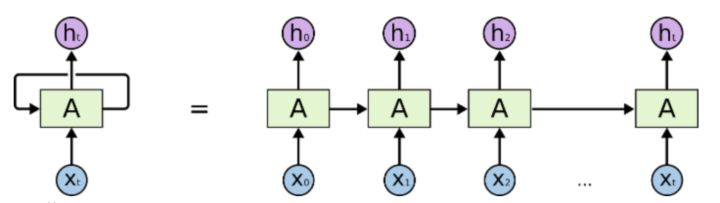上面这张图算是最最最经典的也是最一般的RNN网络结构了。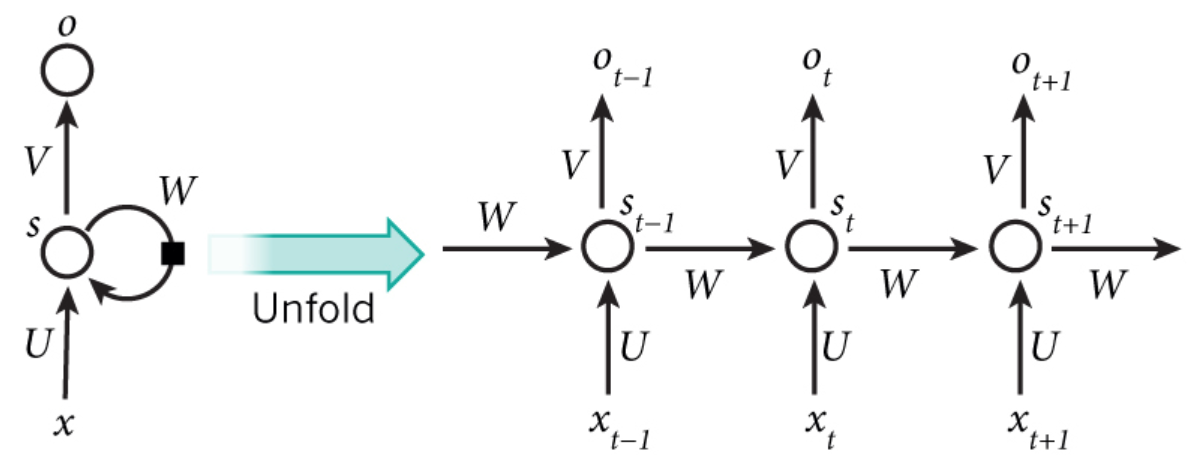因为要讲解步骤，所以用这张图来表示步骤，上图其实是把RNN给展开成我们熟悉的全连接神经网络了，这样我们会比较熟悉。步骤如下：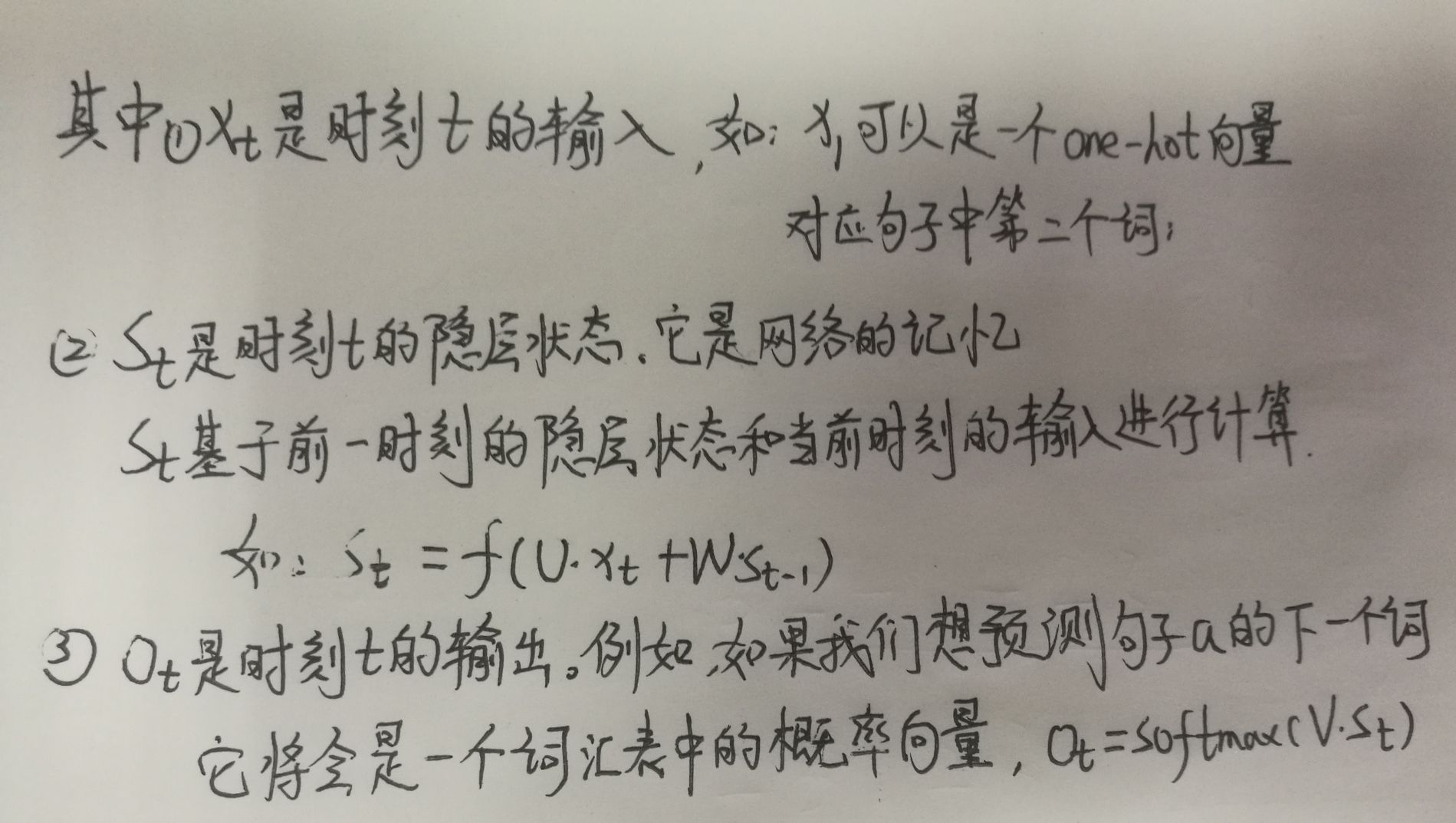基于上述步骤可实现一简单的RNN神经网络，核心流程如下demo所示：

import numpy as np
import os
os.environ["TF_CPP_MIN_LOG_LEVEL"] = "2"

X = [1, 2]
state = [0.0, 0.0]
# 定义不同输入部分的权重
w_cell_state = np.asarray([[0.1, 0.2], [0.3, 0.4]])
w_cell_input = np.asarray([0.5, 0.6])
b_cell = np.asarray([0.1, -0.1])
# 定义输出层的权重
w_output = np.asarray([[0.1], [0.2]])
b_output = 0.1
# 按照时间顺序执行循环神经网络的前向传播过程
for i in range(len(X)):
before_activation = np.dot(state, w_cell_state) + X[i]*w_cell_input+b_cell
state = np.tanh(before_activation)
# 计算当前时刻的最终输出
final_output = np.dot(state, w_output) + b_output
# 输出每一时刻的信息
print("before_activation", before_activation)
print("state", state)
print("final_output", final_output)

只说这个最简单的网络，肯定是没有意思的！所以接下来我会分享一个用RNN来识别手写字体的demo。

2. RNN神经网络识别手写字体

import tensorflow as tf
from tensorflow.examples.tutorials.mnist import input_data
from tensorflow.contrib import rnn
import os
os.environ["TF_CPP_MIN_LOG_LEVEL"] = "2"

# 载入数据

# 设置模型的参数
# 对应输入图片的宽
n_inputs = 28
# 对应输入图片的行
max_time = 28
# 隐藏单元，实质上不是神经元，即LSTM单元
lstm_size = 100
# 图片总共有10个分类
n_classes = 10
# 每批次总共有50个样本
batch_size = 50
n_batch = mnist.train.num_examples//batch_size

# 这里的None=50
x = tf.placeholder(tf.float32, [None, 784])
y = tf.placeholder(tf.float32, [None, 10])

# 初始化权值
weights = tf.Variable(tf.truncated_normal([lstm_size, n_classes], stddev=0.1))
# 初始化偏置值
biases = tf.Variable(tf.constant(0.1, shape=[n_classes]))

# 定义RNN网络
def RNN(X, weights, biases):
inputs = tf.reshape(X, [-1, max_time, n_inputs])
lstm_cell = rnn.BasicLSTMCell(lstm_size)
# outputs维度为shape=(?, 28, 100)；final_state维度为shape=(?, 100)
outputs, final_state = tf.nn.dynamic_rnn(lstm_cell, inputs, dtype=tf.float32)
print("shape(outputs)", outputs)
print("shape(final_state)", final_state)
# 如果使用Seq2Seq模型，则调用outputs；反之则使用final_state
results = tf.nn.softmax(tf.matmul(final_state, weights)+biases)
return results

# 计算RNN的返回结果
prediction = RNN(x, weights, biases)
# 损失函数--交叉熵
cross_entropy = tf.reduce_mean(tf.nn.softmax_cross_entropy_with_logits_v2(labels=y, logits=prediction))
# 结果放在一个布尔型列表中
crroect_prediction = tf.equal(tf.argmax(y, 1), tf.argmax(prediction, 1))
# 求准确率
accuracy = tf.reduce_mean(tf.cast(crroect_prediction, tf.float32))

# 开始训练模型
with tf.Session() as sess:
sess.run(tf.global_variables_initializer())
for epoch in range(10):
for batch in range(n_batch):
batch_xs, batch_ys = mnist.train.next_batch(batch_size)
sess.run(train_op, feed_dict={x: batch_xs, y:batch_ys})
acc = sess.run(accuracy, feed_dict={x: mnist.test.images, y: mnist.test.labels})
print("Iter "+str(epoch)+", Testing Accuracy="+str(acc))

这个demo里有处地方我需要强调一下：

outputs, final_state = tf.nn.dynamic_rnn(lstm_cell, inputs, dtype=tf.float32)
# 如果使用Seq2Seq模型，则调用outputs；反之则使用final_state
results = tf.nn.softmax(tf.matmul(final_state, weights)+biases)

这里面outputs维度为shape=(?, 28, 100)；final_state维度为shape=(?, 100)，具体结构如下图所示：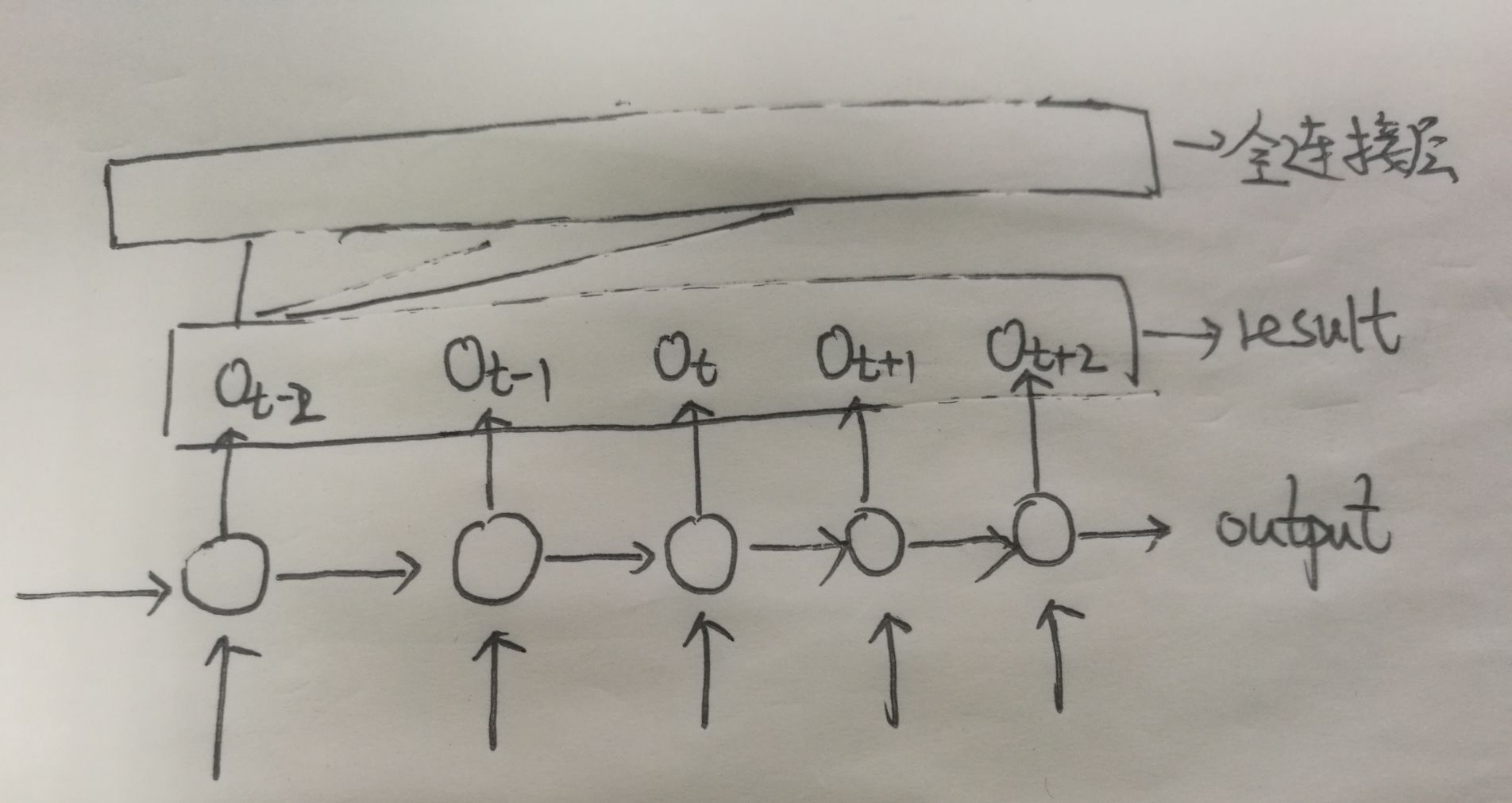在这个demo里我们是没用到output这个LSTM cell的，不是说它没用哈，而是在后面提到的Seq2Seq模型中会用到这个值。

3. RNN神经网络预测下一个字符

不知道大家发现没有，上面讲到的这个最基础的RNN模型中输入数据的“维度”与输出数据的“维度”是一一对应的，即Xt与Ot是相对应的。但是在实际应用中这种情形太少了，跟多的是N->M的关系，即输入数据的维度”与输出数据的“维度”是不同的。比如在机器翻译应用中，将一段汉语翻译成英文。汉语是：我英语不好，翻译成英文是：my English is poor 此处N=5,M=4明显是N与M不相等的情形，此时我们要怎么解决这个模型呢？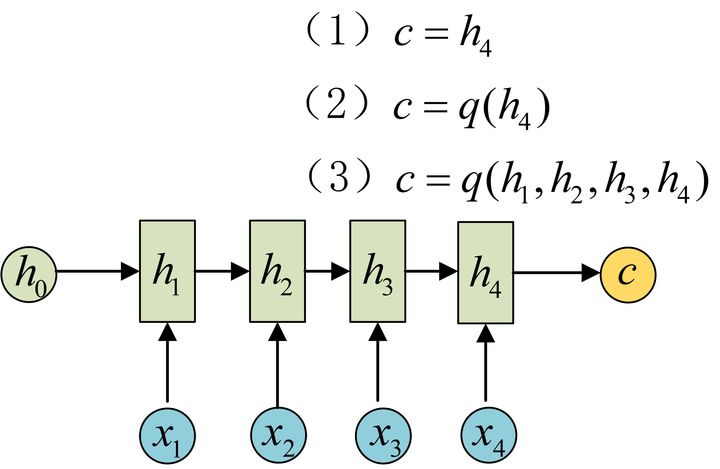那问题就来了，此时我们要怎么解决这个模型呢？

为此得引入一种新的模型，这种结构又叫Encoder-Decoder模型，也可以称之为Seq2Seq模型。如下图所示：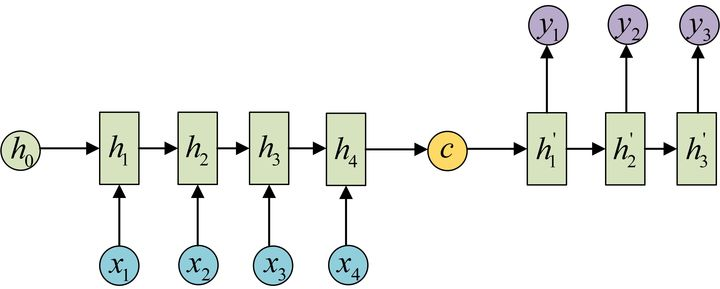还有一种做法是将c当做每一步的输入：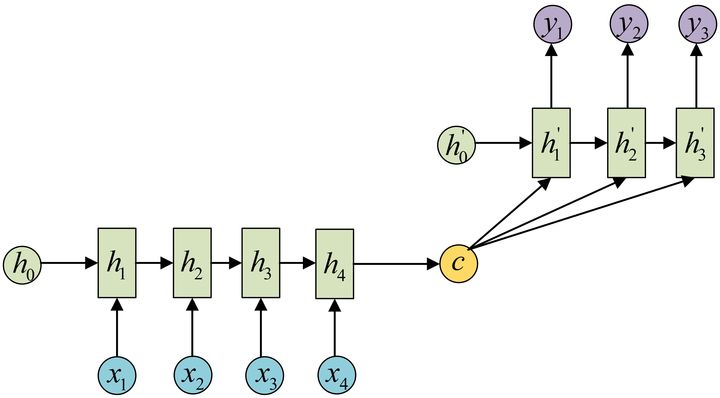按照这个模型，我接下来将会给出能预测下一个字符的RNN模型

import tensorflow as tf
from tensorflow import contrib
import numpy as np
import os
os.environ["TF_CPP_MIN_LOG_LEVEL"] = "2"

sample = "这是一个基于tensorflow的RNN短句子练习"
# 去重放入列表中
idx2char = list(set(sample))
print("idx2char", idx2char)
# 转换为字典，其中把字母作键，索引作为值
char2idx = {c: i for i, c in enumerate(idx2char)}
# 在字典里取出对应值，因为在idx2char中原sample句子的顺序已经被打乱
sample_idx = [char2idx[c] for c in sample]
x_data = [sample_idx[:-1]]
y_data = [sample_idx[1:]]

# 设置该模型的一些参数
dic_size = len(char2idx)
rnn_hidden_size = len(char2idx)
num_classes = len(char2idx)
batch_size = 1
sequence_length = len(sample) - 1

X = tf.placeholder(tf.int32, [None, sequence_length])
Y = tf.placeholder(tf.int32, [None, sequence_length])
# 将input转化为one-hot类型数据输出，此时X的维度变为[None, sequence_length, num_classes]
X_one_hot = tf.one_hot(X, num_classes)

cell = tf.contrib.rnn.BasicLSTMCell(num_units=rnn_hidden_size, state_is_tuple=True)
initial_state = cell.zero_state(batch_size, tf.float32)
outputs, states = tf.nn.dynamic_rnn(cell, X_one_hot, initial_state=initial_state, dtype=tf.float32)
# 加一层全连接层，相当于加一层深度，使预测更准确
outputs = contrib.layers.fully_connected(inputs=outputs, num_outputs=num_classes, activation_fn=None)
print("outputs", tf.shape(outputs))
weights = tf.ones([batch_size, sequence_length])
# 此处包装了encoder和decoder
sequence_loss = tf.contrib.seq2seq.sequence_loss(logits=outputs, targets=Y, weights=weights)
loss = tf.reduce_mean(sequence_loss)

prediction = tf.argmax(outputs, axis=2)

with tf.Session() as sess:
sess.run(tf.global_variables_initializer())
for i in range(3000):
l, _ = sess.run([loss, train], feed_dict={X: x_data, Y: y_data})
result = sess.run(prediction, feed_dict={X: x_data})
# print char using dic
result_str = [idx2char[c] for c in np.squeeze(result)]
print(i, "loss:", l, "Prediction:", "".join(result_str))
print(len(result_str))

模型Run起来的结果如下：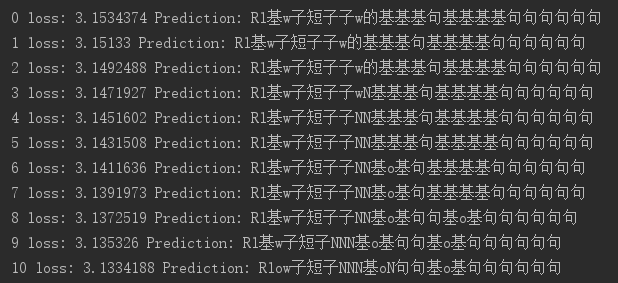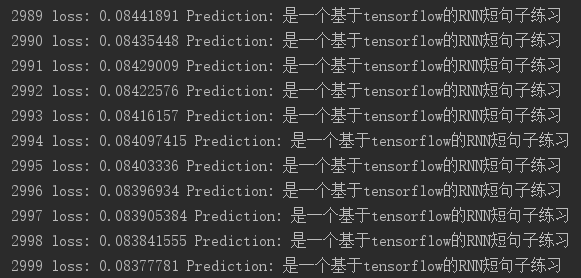可以看到：效果还是不错的！暂时就分享到这儿了，有想到更好的东西再来补充！

展开全文python RNN循环神经网络
• 将神经网络模型训练好之后，在输入层给定一个x，通过网络之后就能够在输出层得到特定的y，那么既然有了这么强大的模型，为什么还需要RNN循环神经网络）呢？ 1 为什么需要RNN循环神经网络） 他们都只能单独的取...

一、获取代码方式

获取代码方式1：
完整代码已上传我的资源：【预测模型】基于matlab RNN循环神经网络预测【含Matlab源码 363期】

获取代码方式2：
通过订阅紫极神光博客付费专栏，凭支付凭证，私信博主，可获得此代码。

备注：
订阅紫极神光博客付费专栏，可免费获得1份代码（有效期为订阅日起，三天内有效）；

二、RNN循环神经网络简介

神经网络可以当做是能够拟合任意函数的黑盒子，只要训练数据足够，给定特定的x，就能得到希望的y，结构图如下：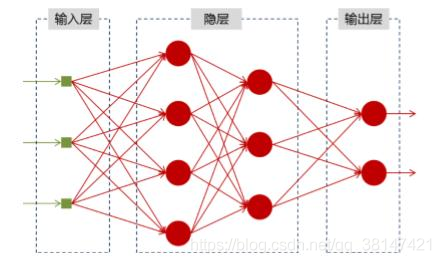将神经网络模型训练好之后，在输入层给定一个x，通过网络之后就能够在输出层得到特定的y，那么既然有了这么强大的模型，为什么还需要RNN（循环神经网络）呢？

1 为什么需要RNN（循环神经网络）
他们都只能单独的取处理一个个的输入，前一个输入和后一个输入是完全没有关系的。但是，某些任务需要能够更好的处理序列的信息，即前面的输入和后面的输入是有关系的。

比如，当我们在理解一句话意思时，孤立的理解这句话的每个词是不够的，我们需要处理这些词连接起来的整个序列； 当我们处理视频的时候，我们也不能只单独的去分析每一帧，而要分析这些帧连接起来的整个序列。
以nlp的一个最简单词性标注任务来说，将我 吃 苹果 三个单词标注词性为 我/nn 吃/v 苹果/nn。
那么这个任务的输入就是：
我 吃 苹果 （已经分词好的句子）
这个任务的输出是：
我/nn 吃/v 苹果/nn(词性标注好的句子)
对于这个任务来说，我们当然可以直接用普通的神经网络来做，给网络的训练数据格式了就是我-> 我/nn 这样的多个单独的单词->词性标注好的单词。
但是很明显，一个句子中，前一个单词其实对于当前单词的词性预测是有很大影响的，比如预测苹果的时候，由于前面的吃是一个动词，那么很显然苹果作为名词的概率就会远大于动词的概率，因为动词后面接名词很常见，而动词后面接动词很少见。
所以为了解决一些这样类似的问题，能够更好的处理序列的信息，RNN就诞生了。
2 RNN结构
首先看一个简单的循环神经网络如，它由输入层、一个隐藏层和一个输出层组成：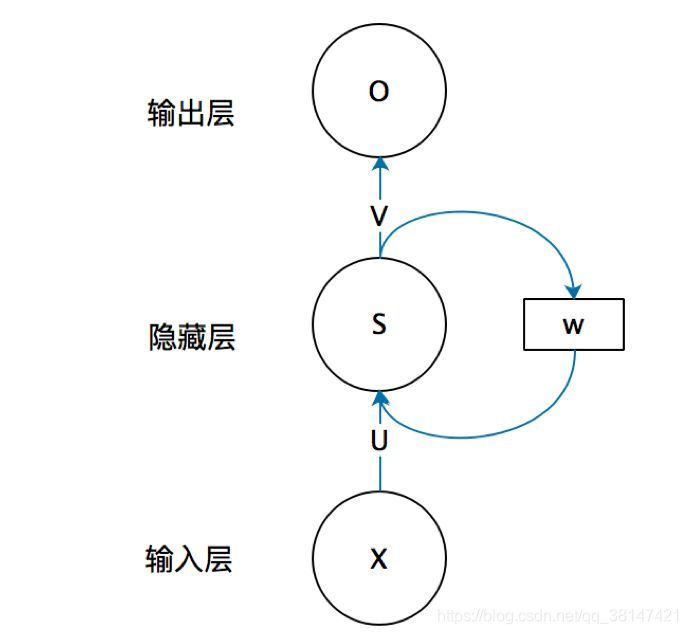不知道初学的同学能够理解这个图吗，反正我刚开始学习的时候是懵逼的，每个结点到底代表的是一个值的输入，还是说一层的向量结点集合，如何隐藏层又可以连接到自己，等等这些疑惑~这个图是一个比较抽象的图。
我们现在这样来理解，如果把上面有W的那个带箭头的圈去掉，它就变成了最普通的全连接神经网络。x是一个向量，它表示输入层的值（这里面没有画出来表示神经元节点的圆圈）；s是一个向量，它表示隐藏层的值（这里隐藏层面画了一个节点，你也可以想象这一层其实是多个节点，节点数与向量s的维度相同）；
U是输入层到隐藏层的权重矩阵，o也是一个向量，它表示输出层的值；V是隐藏层到输出层的权重矩阵。
那么，现在我们来看看W是什么。循环神经网络的隐藏层的值s不仅仅取决于当前这次的输入x，还取决于上一次隐藏层的值s。权重矩阵 W就是隐藏层上一次的值作为这一次的输入的权重。
我们给出这个抽象图对应的具体图：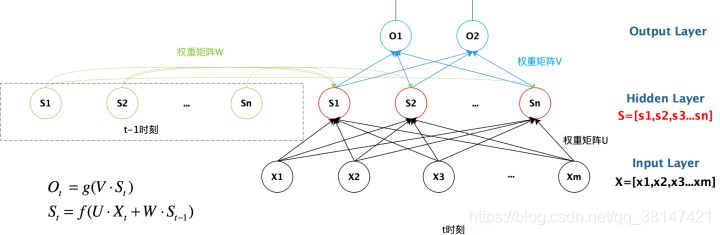我们从上图就能够很清楚的看到，上一时刻的隐藏层是如何影响当前时刻的隐藏层的。
如果我们把上面的图展开，循环神经网络也可以画成下面这个样子：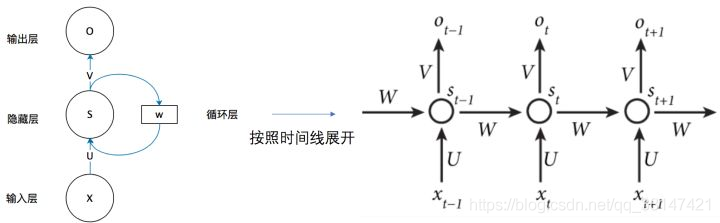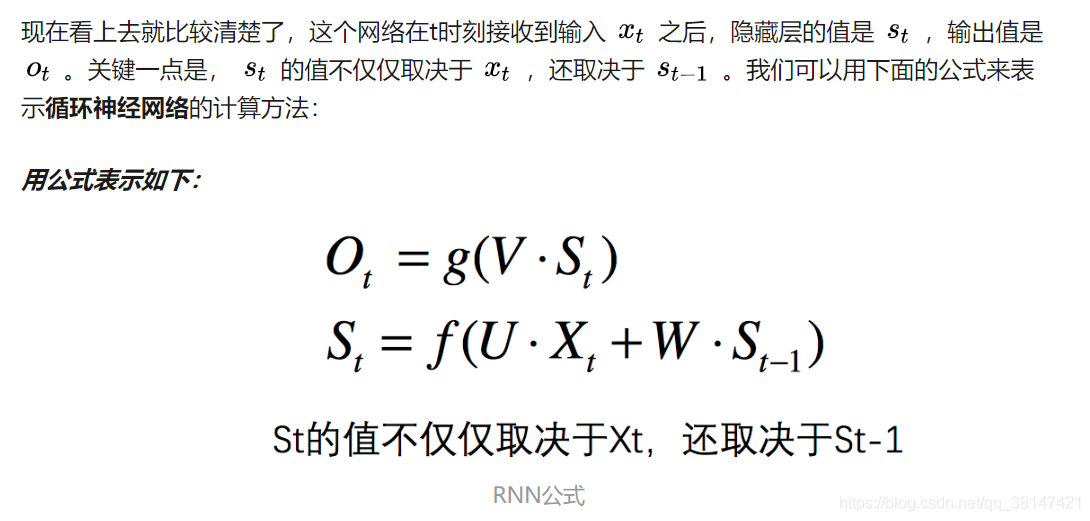3 总结
好了，到这里大概讲解了RNN最基本的几个知识点，能够帮助大家直观的感受RNN和了解为什么需要RNN，后续总结它的反向求导知识点。
最后给出RNN的总括图：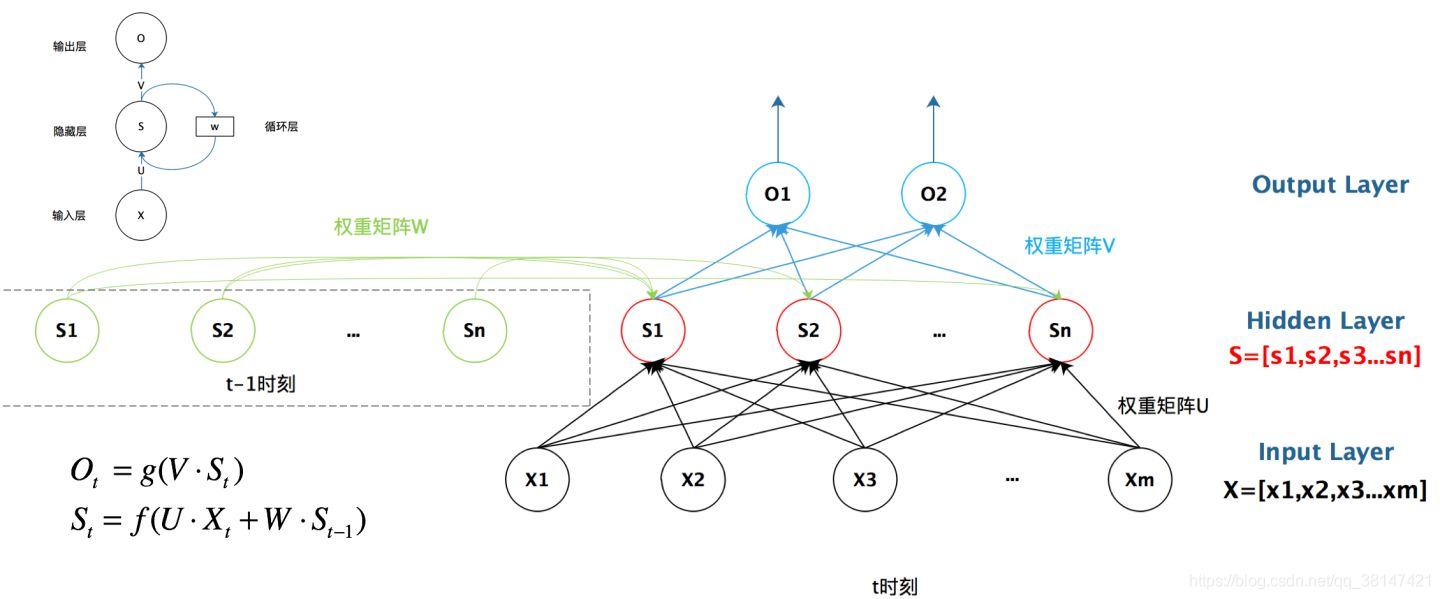三、部分源代码

clc;
clear all;
[train_data,test_data]=LSTM_data_process();
data_length=size(train_data,1);
data_num=size(train_data,2);
%% 网络参数初始化
% 结点数设置
input_num=data_length;
cell_num=5;
output_num=size(test_data,1);
% 网络中门的偏置
bias_input_gate=rand(1,cell_num);
bias_forget_gate=rand(1,cell_num);
bias_output_gate=rand(1,cell_num);
%网络权重初始化
ab=20;
weight_input_x=rand(input_num,cell_num)/ab;
weight_input_h=rand(output_num,cell_num)/ab;
weight_inputgate_x=rand(input_num,cell_num)/ab;
weight_inputgate_c=rand(cell_num,cell_num)/ab;
weight_forgetgate_x=rand(input_num,cell_num)/ab;
weight_forgetgate_c=rand(cell_num,cell_num)/ab;
weight_outputgate_x=rand(input_num,cell_num)/ab;
weight_outputgate_c=rand(cell_num,cell_num)/ab;
%hidden_output权重
weight_preh_h=rand(cell_num,output_num);
%网络状态初始化
cost_gate=0.25;
h_state=rand(output_num,data_num);
cell_state=rand(cell_num,data_num);
%% 网络训练学习
for iter=1:100
yita=0.01;            %每次迭代权重调整比例
for m=1:data_num
%前馈部分
if(m==1)
gate=tanh(train_data(:,m)'*weight_input_x);
input_gate_input=train_data(:,m)'*weight_inputgate_x+bias_input_gate;
output_gate_input=train_data(:,m)'*weight_outputgate_x+bias_output_gate;
for n=1:cell_num
input_gate(1,n)=1/(1+exp(-input_gate_input(1,n)));
output_gate(1,n)=1/(1+exp(-output_gate_input(1,n)));
end
forget_gate=zeros(1,cell_num);
forget_gate_input=zeros(1,cell_num);
cell_state(:,m)=(input_gate.*gate)';
else
gate=tanh(train_data(:,m)'*weight_input_x+h_state(:,m-1)'*weight_input_h);
input_gate_input=train_data(:,m)'*weight_inputgate_x+cell_state(:,m-1)'*weight_inputgate_c+bias_input_gate;
forget_gate_input=train_data(:,m)'*weight_forgetgate_x+cell_state(:,m-1)'*weight_forgetgate_c+bias_forget_gate;
output_gate_input=train_data(:,m)'*weight_outputgate_x+cell_state(:,m-1)'*weight_outputgate_c+bias_output_gate;
for n=1:cell_num
input_gate(1,n)=1/(1+exp(-input_gate_input(1,n)));
forget_gate(1,n)=1/(1+exp(-forget_gate_input(1,n)));
output_gate(1,n)=1/(1+exp(-output_gate_input(1,n)));
end
cell_state(:,m)=(input_gate.*gate+cell_state(:,m-1)'.*forget_gate)';
end
pre_h_state=tanh(cell_state(:,m)').*output_gate;
h_state(:,m)=(pre_h_state*weight_preh_h)';
end
% 误差的计算
Error=h_state(:,m)-train_data(:,m);
%     Error=h_state(:,:)-train_data(end,:);
Error_Cost(1,iter)=sum(Error.^2);
if Error_Cost(1,iter) < cost_gate
iter
break;
end
[ weight_input_x,...
weight_input_h,...
weight_inputgate_x,...
weight_inputgate_c,...
weight_forgetgate_x,...
weight_forgetgate_c,...
weight_outputgate_x,...
weight_outputgate_c,...
weight_preh_h ]=LSTM_updata_weight(m,yita,Error,...
weight_input_x,...
weight_input_h,...
weight_inputgate_x,...
weight_inputgate_c,...
weight_forgetgate_x,...
weight_forgetgate_c,...
weight_outputgate_x,...
weight_outputgate_c,...
weight_preh_h,...
cell_state,h_state,...
input_gate,forget_gate,...
output_gate,gate,...
train_data,pre_h_state,...
input_gate_input,...
output_gate_input,...
forget_gate_input);

end

四、运行结果五、matlab版本及参考文献

1 matlab版本
2014a

2 参考文献
 包子阳,余继周,杨杉.智能优化算法及其MATLAB实例（第2版）[M].电子工业出版社，2016.
张岩,吴水根.MATLAB优化算法源代码[M].清华大学出版社，2017.
周品.MATLAB 神经网络设计与应用[M].清华大学出版社，2013.
陈明.MATLAB神经网络原理与实例精解[M].清华大学出版社，2013.
方清城.MATLAB R2016a神经网络设计与应用28个案例分析[M].清华大学出版社，2018.

展开全文rnn matlab 神经网络
• 上图一个单词一个线性层的model,当单词数量变多时,参数量会变的很大 权值共享后只需要一个线性层 具有时间概念 RNN-Model 数学形式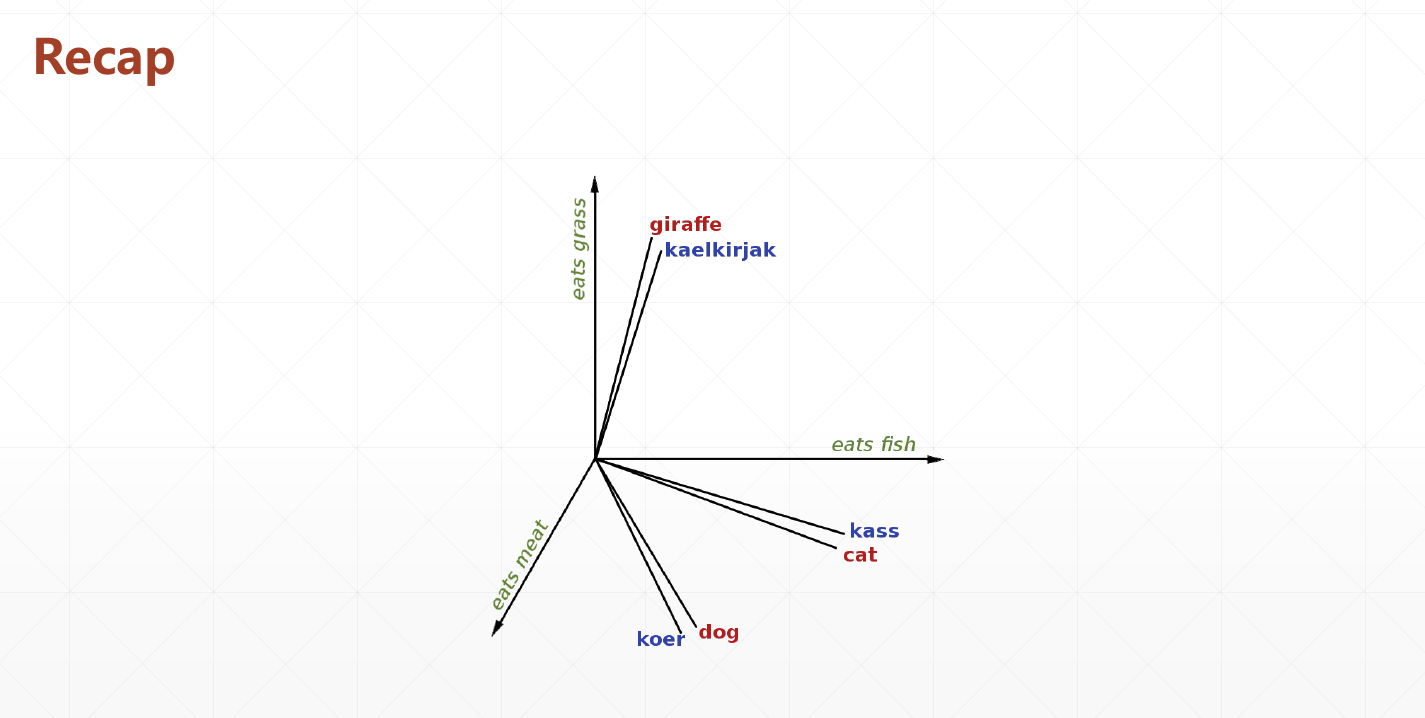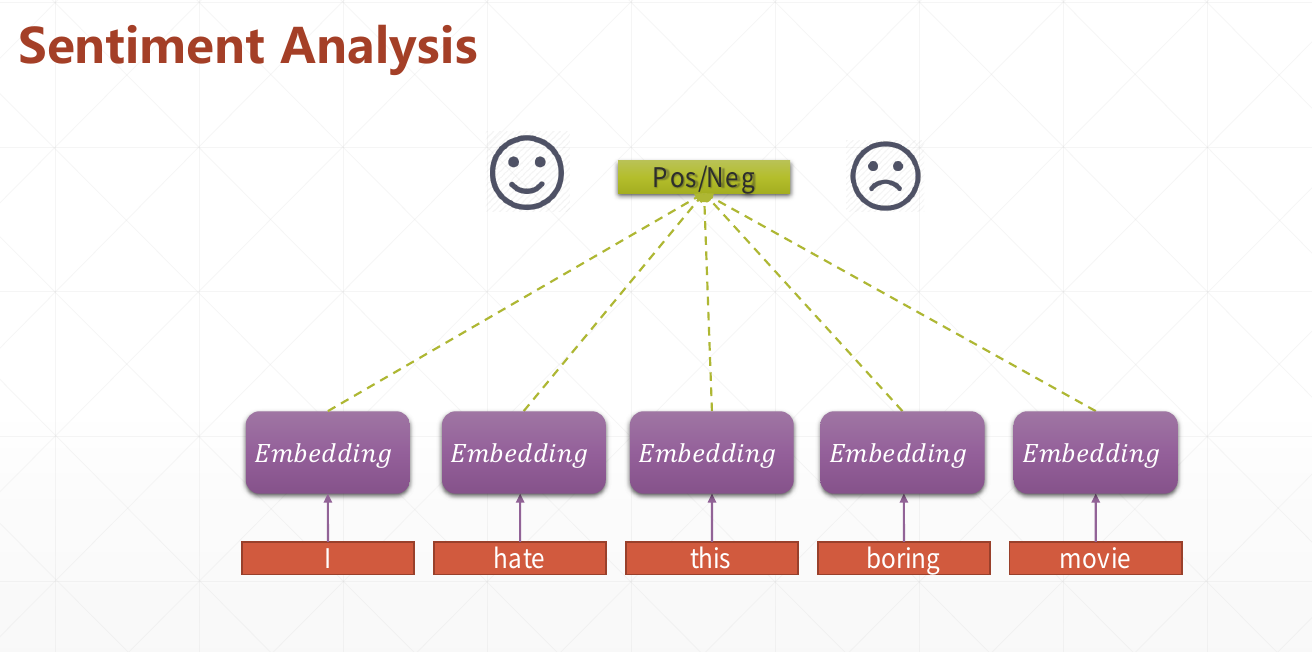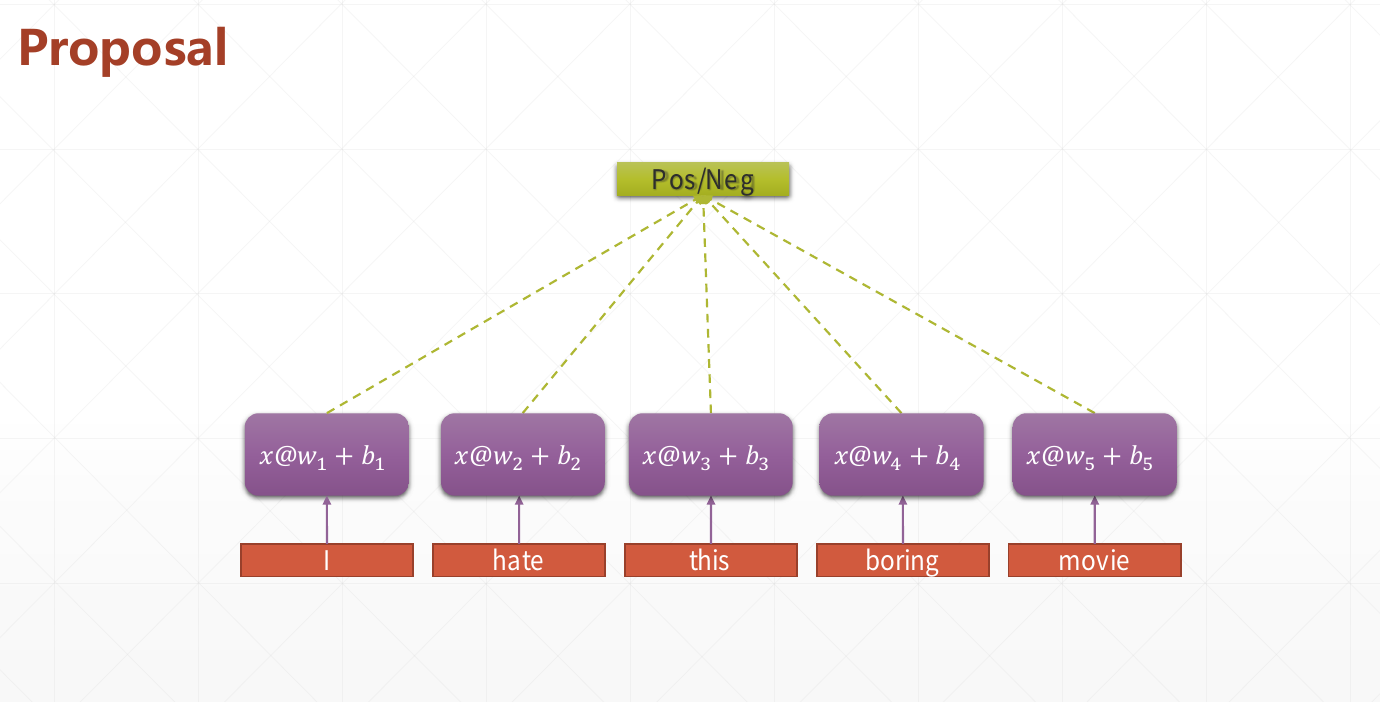上图一个单词一个线性层的model,当单词数量变多时,参数量会变的很大权值共享后只需要一个线性层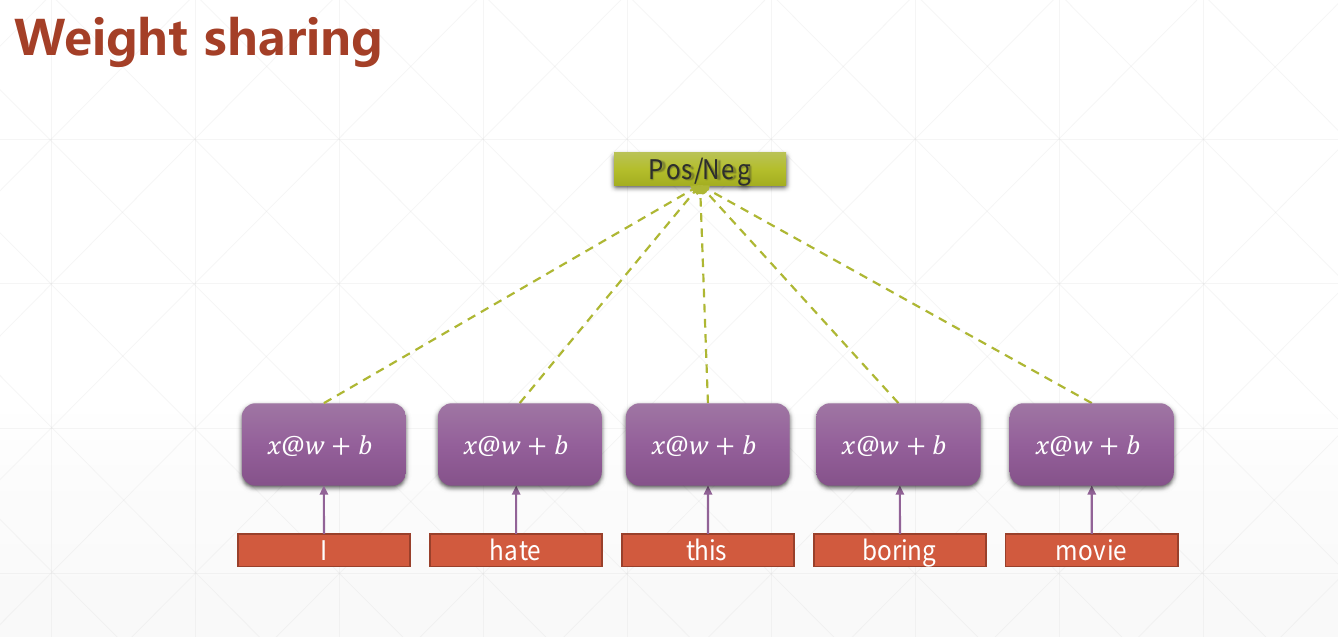具有时间概念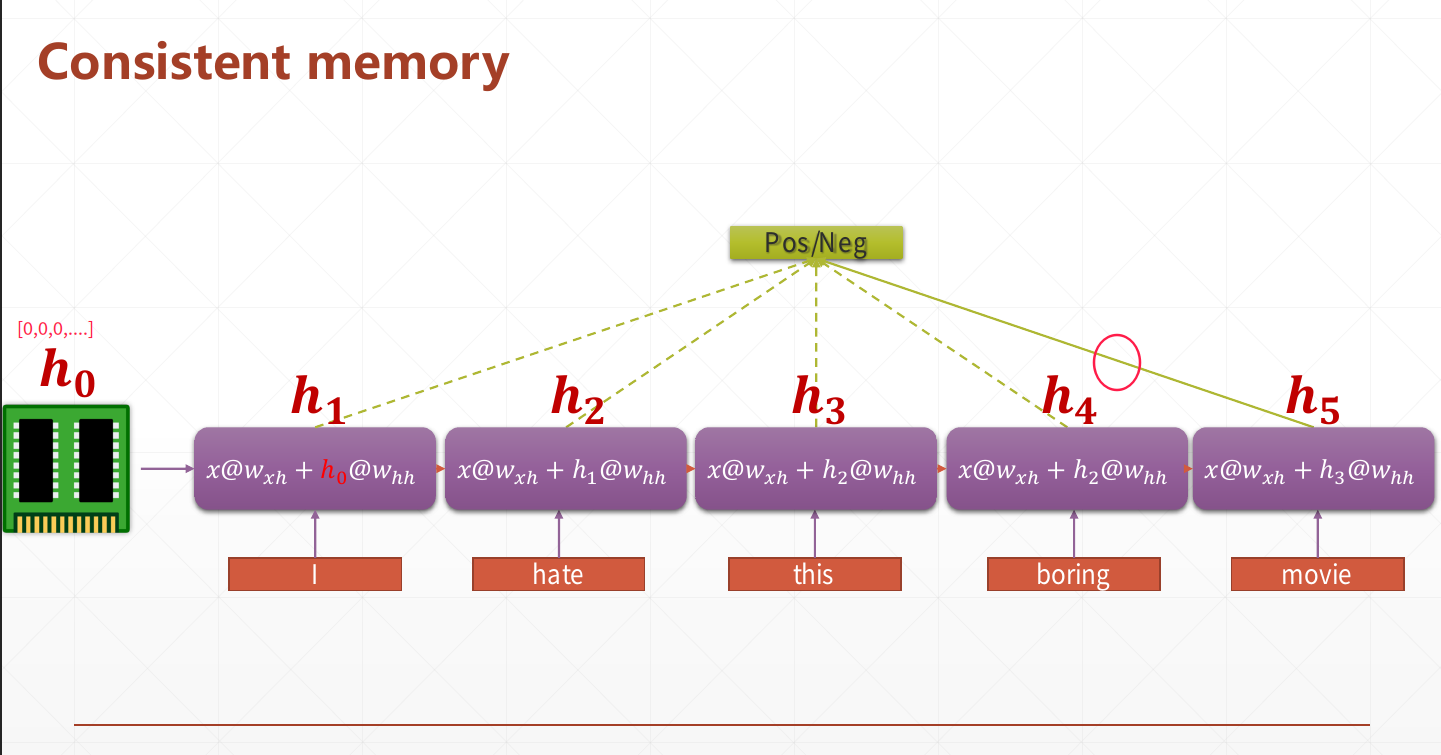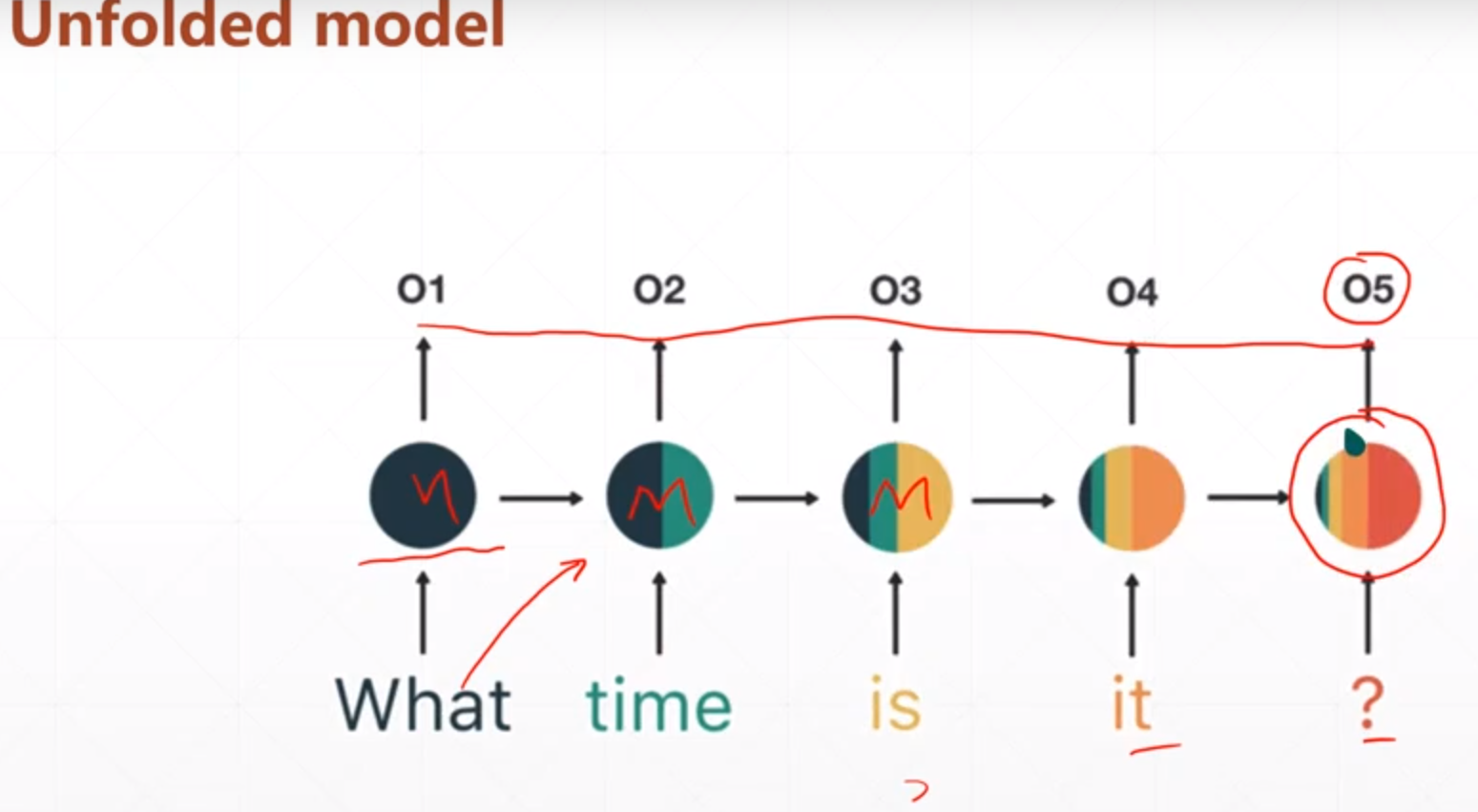RNN-Model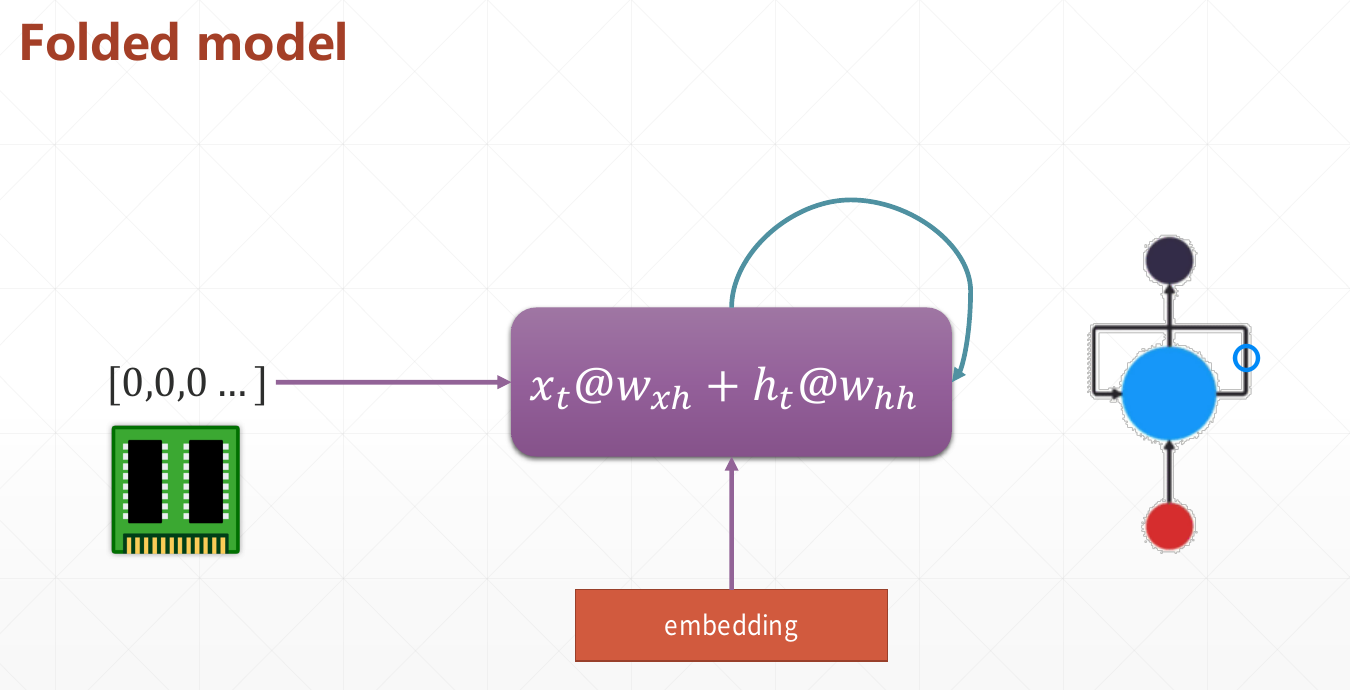数学形式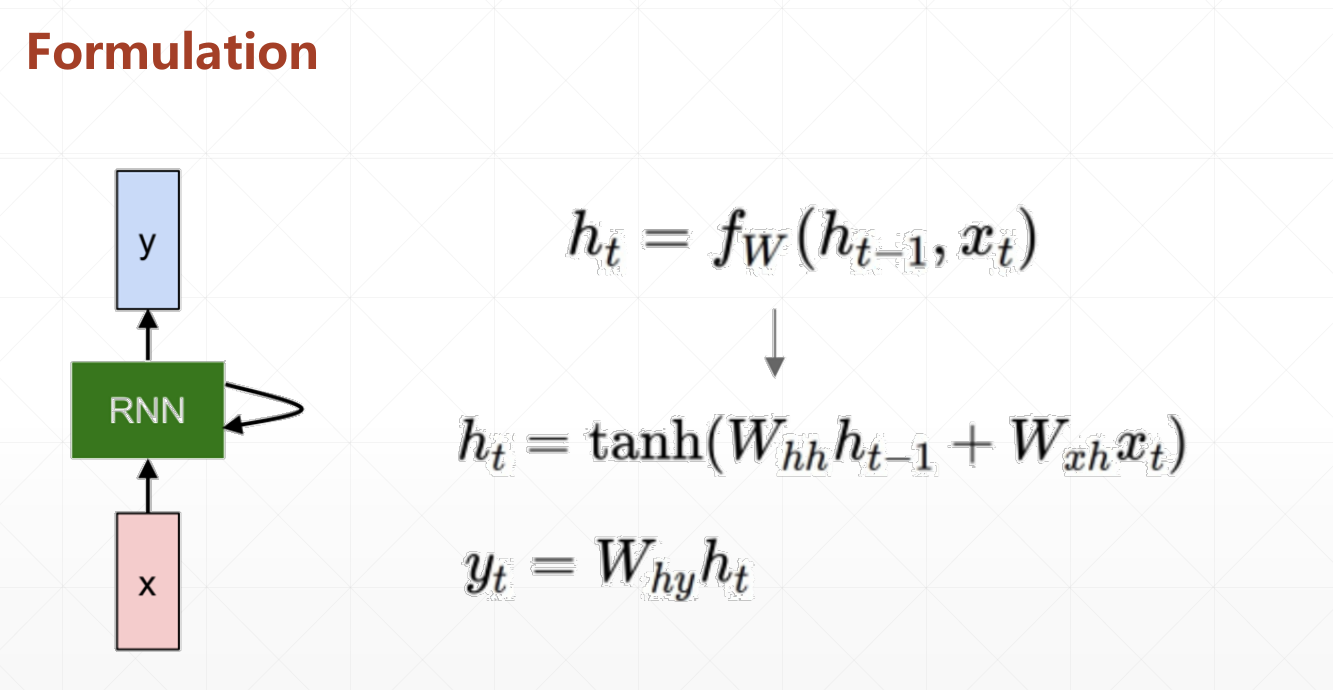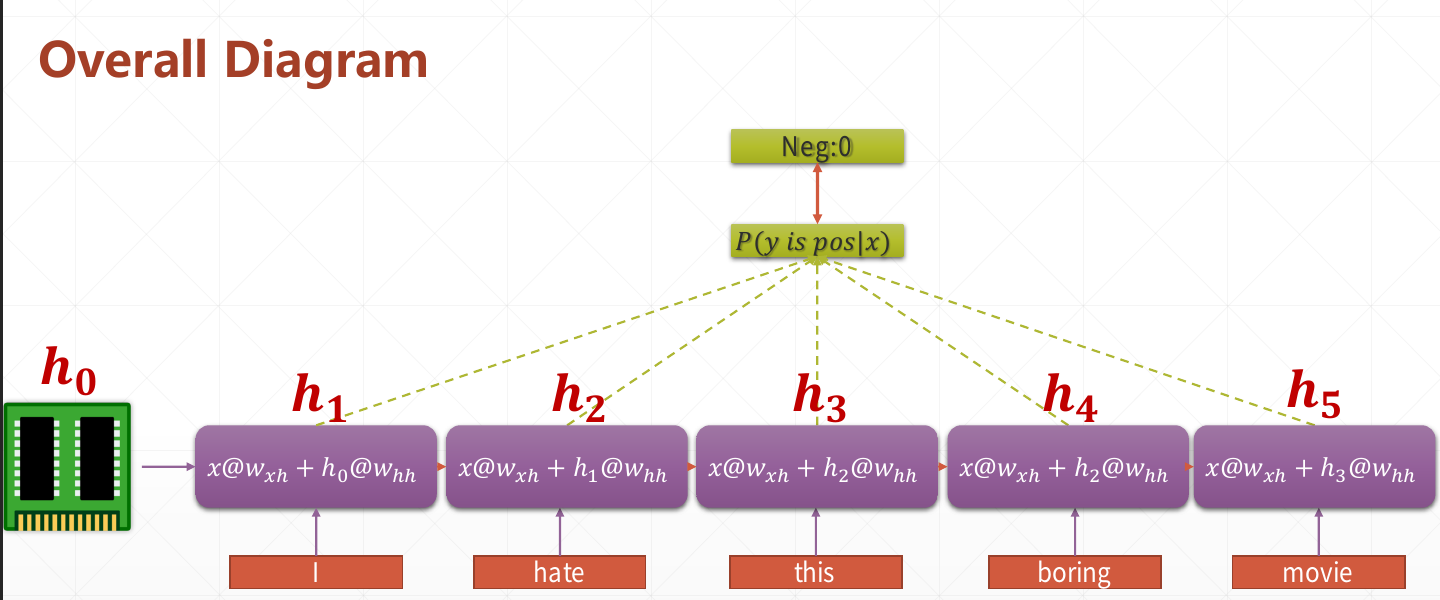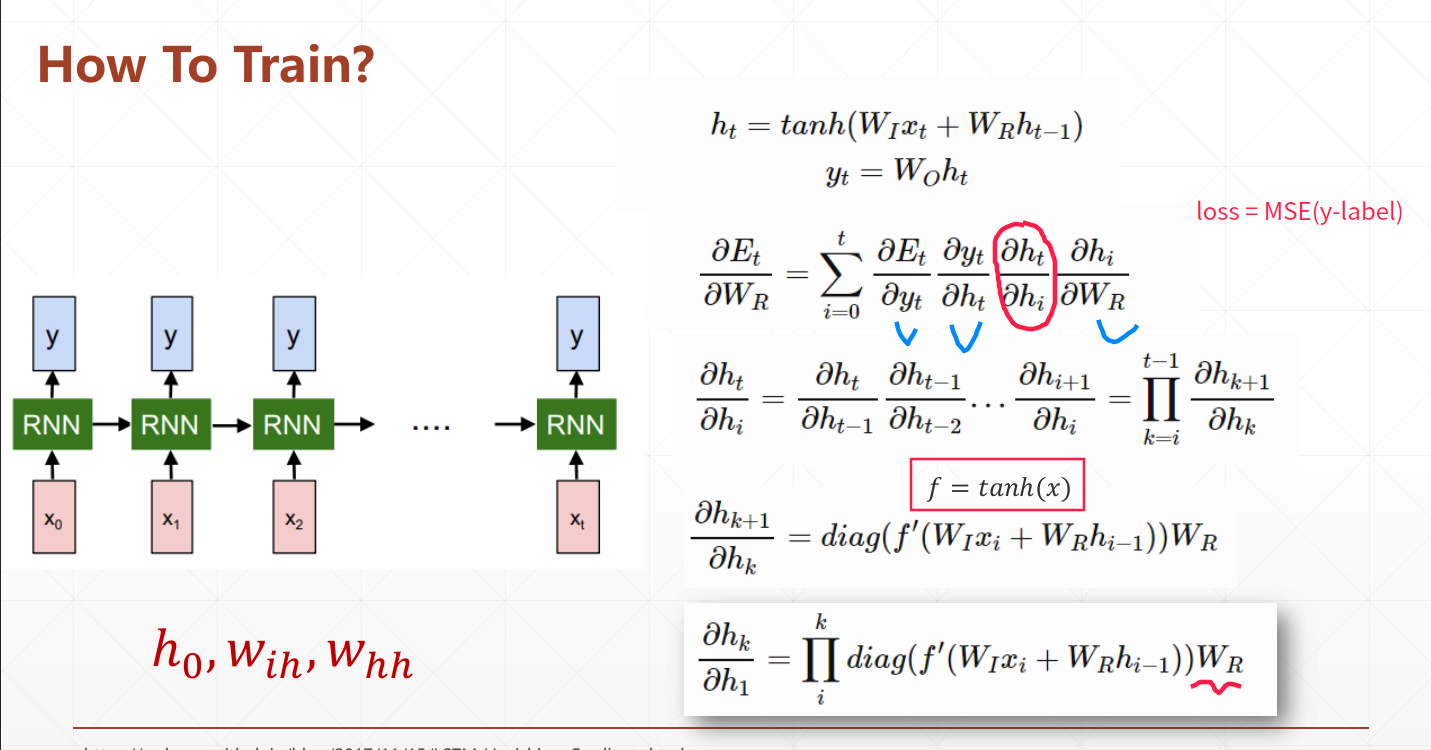在时间维度上展开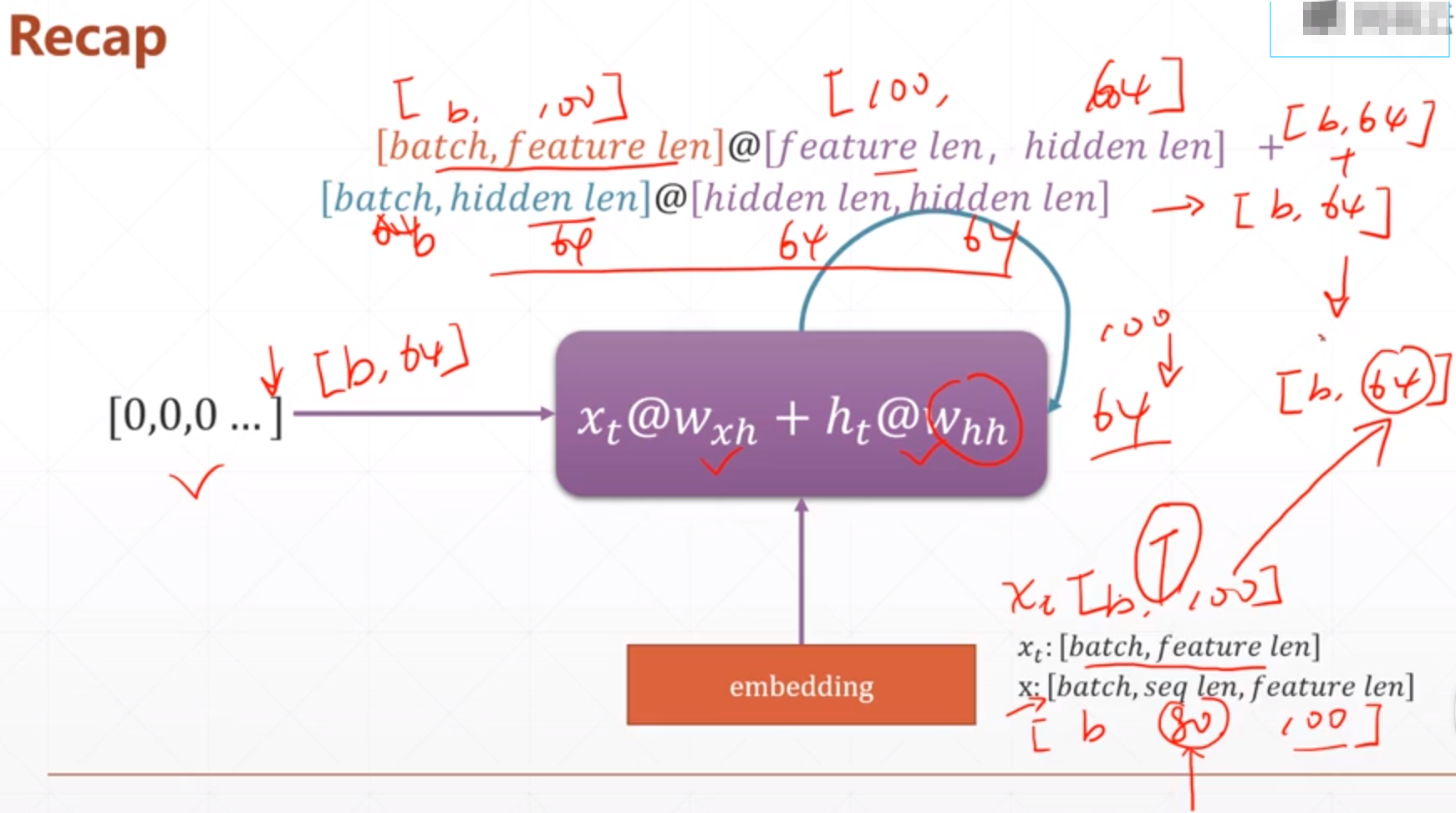In : cell = layers.SimpleRNNCell(3)  # 在时间维度上进行一次前向运算叫做一个cell

In : cell.build(input_shape=(None, 4))

In : cell.trainable_variables
Out:
[<tf.Variable 'kernel:0' shape=(4, 3) dtype=float32, numpy=
array([[-0.62658715,  0.2633381 ,  0.52798474],
[-0.61995786,  0.06983739,  0.5513731 ],
[ 0.38611555, -0.4026158 ,  0.03712767],
[ 0.30363703, -0.17313945, -0.49760813]], dtype=float32)>,
<tf.Variable 'recurrent_kernel:0' shape=(3, 3) dtype=float32, numpy=
array([[-0.7433469 ,  0.27049097, -0.611776  ],
[-0.3911615 , -0.9176911 ,  0.0695377 ],
[ 0.54261214, -0.29099387, -0.7879687 ]], dtype=float32)>,
<tf.Variable 'bias:0' shape=(3,) dtype=float32, numpy=array([0., 0., 0.], dtype=float32)>]

In : # 'kernel' 是Wxh 'recurrent_kernel' 是Whh

Single layer RNN Cell

In : x = tf.random.normal([4, 80, 100])

In : xt0 = x[:,0,:] # 第一个时间戳

In : cell = tf.keras.layers.SimpleRNNCell(64)

In : out, ht1 = cell(xt0, [tf.zeros([4, 64])])

In : out.shape, ht1.shape  # ht1 = [out]
Out: (TensorShape([4, 64]), TensorShape([4, 64]))

In : id(out), id(ht1)
Out: (140385529293816, 140385529293816)

W, b

In : cell = layers.SimpleRNNCell(64)

In : cell.build(input_shape=(None, 100))

In : cell.trainable_variables
Out:
[<tf.Variable 'kernel:0' shape=(100, 64) dtype=float32, numpy=
array([[-0.0462963 ,  0.18592049, -0.07400571, ...,  0.02941154,
-0.05667783,  0.17601068],
[ 0.02097672, -0.06260185,  0.07964225, ..., -0.11110415,
-0.01080528, -0.00902247],
[-0.05255362,  0.07717623, -0.11303762, ..., -0.05901927,
-0.03984712, -0.10676748],
...,
[-0.10032847,  0.13400845, -0.09042987, ..., -0.05995423,
0.01902728, -0.18547705],
[-0.1514959 ,  0.03897026, -0.01399444, ..., -0.01285194,
-0.02200188, -0.08484551],
[ 0.18662949,  0.12275948, -0.0774735 , ...,  0.16830678,
0.11057346,  0.04651706]], dtype=float32)>,
<tf.Variable 'recurrent_kernel:0' shape=(64, 64) dtype=float32, numpy=
array([[-0.03017783,  0.00358709,  0.10952011, ...,  0.13098714,
-0.01387545, -0.04514761],
[ 0.05304666, -0.12605345,  0.01283378, ..., -0.15707201,
0.00664928,  0.12498017],
[-0.11184912, -0.04400529, -0.12750888, ...,  0.12323189,
0.03086963, -0.06987697],
...,
[ 0.0790165 ,  0.18564737,  0.00124895, ..., -0.08405672,
-0.16828664,  0.21780132],
[-0.18108547,  0.03274943, -0.08180425, ..., -0.0022919 ,
-0.00342036, -0.10432611],
[-0.2050607 ,  0.04017716, -0.15770806, ...,  0.00416686,
-0.15942793,  0.22969675]], dtype=float32)>,
<tf.Variable 'bias:0' shape=(64,) dtype=float32, numpy=
array([0., 0., 0., 0., 0., 0., 0., 0., 0., 0., 0., 0., 0., 0., 0., 0., 0.,
0., 0., 0., 0., 0., 0., 0., 0., 0., 0., 0., 0., 0., 0., 0., 0., 0.,
0., 0., 0., 0., 0., 0., 0., 0., 0., 0., 0., 0., 0., 0., 0., 0., 0.,
0., 0., 0., 0., 0., 0., 0., 0., 0., 0., 0., 0., 0.], dtype=float32)>]

In : # Wxh:(100, 64), Whh:(64, 64), bias:(64, )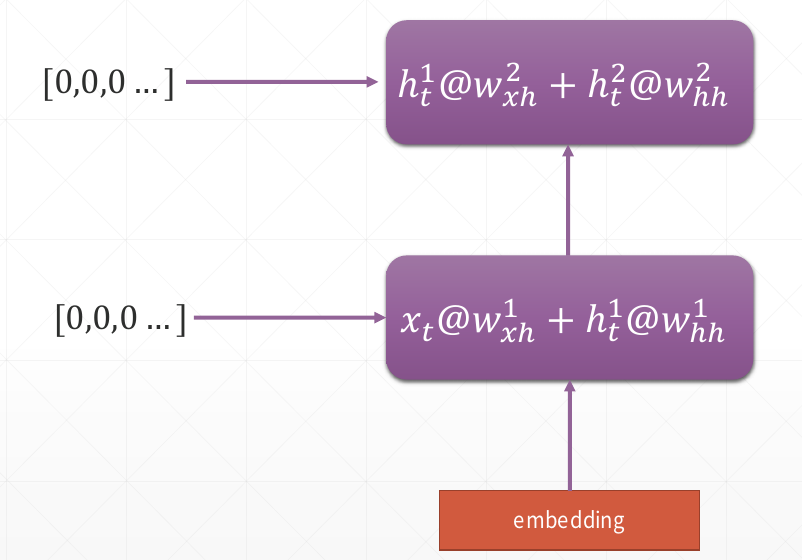In : # Multi-Layers RNN

In : x = tf.random.normal([4, 80, 100])

In : Xt0 = x[:,0,:]

In : cell0 = tf.keras.layers.SimpleRNNCell(64)

In : cell2 = tf.keras.layers.SimpleRNNCell(64)

In : state0 = [tf.zeros([4, 64])]

In : state2 = [tf.zeros([4, 64])]

In : out0, state0 = cell0(Xt0, state0)

In : out2, state2 = cell2(out0, state2)

In : out2.shape, state2.shape
Out: (TensorShape([4, 64]), TensorShape([4, 64]))

Multi-Layers RNN

In : x.shape
Out: TensorShape([4, 80, 100])

In : state0 = [tf.zeros([4, 64])]

In : state1 = [tf.zeros([4, 64])]

In : cell0 = tf.keras.layers.SimpleRNNCell(64)

In : cell1 = tf.keras.layers.SimpleRNNCell(64)

In : for word in tf.unstack(x, axis=1):
...:     # h1 = x * Wxh + h0 * Whh
...:     # out0: [b, 64]
...:     out0, state0 = cell0(word, state0)
...:     # out1: [b, 64]
...:     out1, state1 = cell1(out0, state1)

RNN Layer
自动在时间序列上拆分

# return_sequences = True, 表示返回每个时间戳这一层的state作为下一层的输入
In : rnn = Sequential([layers.SimpleRNN(64, dropout=0.5, return_sequences=True, unroll=True),
...:                   layers.SimpleRNN(64, dropout=0.5, unroll=True)])

In : x.shape
Out: TensorShape([4, 80, 100])

In : x = rnn(x)

In : x.shape
Out: TensorShape([4, 64])

展开全文神经网络 深度学习 tensorflow python 循环神经网络
• RNN循环神经网络简介

千次阅读 2021-03-12 14:32:43
1.RNN 循环神经网络简介 2.LSTM RNN 长短期记忆循环神经网络简介 3.GRU RNN 门循环单元循环神经网络简介 一.RNN 循环神经网络简介 1990年, 美国认知科学家Jeffrey L. Elman 对jordan network进行了简化,并采用BP...

一.RNN 循环神经网络简介

1990年, 美国认知科学家Jeffrey L. Elman 对jordan network进行了简化,并采用BP算法进行训练，便有了如今最简单的包含单个自连接节点的RNN 模型
循环神经网络，是指在全连接神经网络的基础上增加了前后时序上的关系，可以更好地处理比如机器翻译等的与时序相关的问题。
RNN的目的就是用来处理序列数据的。在传统的神经网络模型中，是从输入层到隐含层再到输出层，层与层之间是全连接的，每层之间的节点是无连接的。但是这种普通的神经网络对于很多问题都无能无力。比如你要预测句子的下一个单词是什么，一般需要用到前面的单词，因为一个句子中前后单词并不是独立的。又或者你要预测明天的股票价格，你就需要前段时间的股票价格作为输入。这些都需要每层之间的节点产生关联才能实现。
RNN之所以称为循环神经网路，即一个序列当前的输出与前面的输出也有关。具体的表现形式为网络会对前面的信息进行记忆并应用于当前输出的计算中，即隐藏层之间的节点不再无连接而是有连接的，并且隐藏层的输入不仅包括输入层的输出还包括上一时刻隐藏层的输出。
理论上，RNN能够对任何长度的序列数据进行处理。但是在实践中，为了降低复杂性往往假设当前的状态只与前面的几个状态相关，这也是RNN的局限性，当输入长度过长时，最早的输入可能会在神经网络反向传播时产生梯度爆炸或者梯度消失，导致RNN无法回忆起过于久远的记忆，会降低预测的准确率，于是就有了下面的 LSTM RNN网络。

单个RNN神经元结构：二.LSTM RNN 长短期记忆循环神经网络简介

1997年，人工智能研究所的主任Jurgen Schmidhuber 提出长短期记忆（LSTM），LSTM使用门控单元及记忆机制大大缓解了早期RNN训练的问题。
LSTM RNN 和普通的 RNN 相比，多出了三个控制器（输入门，遗忘门和输出门）以便控制全局的记忆。
可以把 LSTM RNN 理解成一部电影，包含了主线和支线，当主线剧情进行时，如果某个支线对结局十分重要，输入门就会将这个支线按重要程度写入主线，在进行分析。如果此时支线改变了之前主线剧情的想法，那么遗忘门就会将之前某些主线剧情忘记，按比例替换成新支线剧情。最后，输出门会根据当前的主线剧情和支线剧情决定输出结果。
这样即使是经过了很长输入序列的的神经网络，也能记住久远的重要变化。

单个LSTM神经元结构：三.GRU RNN 门循环单元循环神经网络简介

GRU 把 LSTM 中的三个门限（输入门，遗忘门和输出门），变成了两个（更新门和重置门）。
一般来说两者效果差不多，性能在很多任务上也不分伯仲，主要区别在于以下两点。
1.GRU参数更少，收敛更快。
2.LSTM参数比GRU参数多一些，数据量很大时LSTM效果会更好一些。

单个GRU神经元结构：展开全文• 1、RNN的简单介绍        我们前面提到的无论是人工神经网络还是CNN卷积神经网络，他们的前提都是：元素之间是相互独立的，...因此，我们就需要使用循环神经网络了，他的本质是：像算法 人工智能 神经网络
• 深度学习神经网络之循环神经网络RNN）Matlab实现循环神经网络RNN
• 目录 前言 一、普通神经网络 二、循环神经网络 1.前向传播 ...前段时间实验室人手一本《Deep Learning》，本文章结合这本圣经和博客上各类知识以及我自己的理解和实践，针对RNN循环神经网络作出...RNN循环神经网络
• RNN 循环神经网络 (分类) RNN 循环神经网络 (回归) 目录Pytorch教程目录训练数据RNN模型训练全部代码 训练数据 我们要用到的数据就是这样的一些数据, 我们想要用 sin 的曲线预测出 cos 的曲线. import torch from
• #4.2_RNN_循环神经网络_分类_(PyTorch_tutorial_神经网络_教学)学习资源
• RNN递归神经网络的直观理解：基于TensorFlow的简单RNN例子RNN 直观理解 一个非常棒的RNN入门Anyone Can learn To Code LSTM-RNN in Python(Part 1: RNN) 基于此文章，本文给出我自己的一些愚见 基于此文章，给出其中...深度学习 递归神经网络 TensorFlow RNN
• Google TensorFlow程序员...吴恩达deepLearning.ai循环神经网络RNN学习笔记(理论篇) 我们将实现以下结构的RNN，在这个例子中 Tx = Ty。 向量表示以及它的维度 Input with nx number of units 对单个输入样本，x(i)
• 因此，就有了现在的循环神经网络，他的本质是：拥有记忆的能力，并且会根据这些记忆的内容来进行推断。因此，他的输出就依赖于当前的输入和记忆。 网络结构及原理 循环神经网络的基本结构特别简单，就是将网络的输出...
• 一、时序数据 卷积神经网络是针对二维位置...对于按时间轴不停产生的信号，神经网络中，我们称其为temporal signals，而循环神经网络处理的就是拥有时序相关或者前后相关的数据类型（Sequence）。 二、embeddin...IMDB下载 RNN循环神经网络 RNN梯度
• 小白学深度之RNN循环神经网络

千次阅读 多人点赞 2020-07-12 23:08:32
我本身也是个小白，近期在学这方面的内容，这篇博客可以说是一个知识的总结吧。站在小白视角看待RNN，我这么笨都能看明白，相信你们肯定也能明白。希望对大家有所帮助，不足之处还望指出。神经网络 python 深度学习 人工智能
• RNN循环神经网络 (回归)

千次阅读 2019-02-18 15:24:23
我制作的 循环神经网络 RNN 动画简介 我制作的 循环神经网络 LSTM 动画简介 PyTorch 官网 要点  循环神经网络让神经网络有了记忆, 对于序列话的数据,循环神经网络能达到更好的效果. 如果你对循环神经网络还没有...循环神经网络 RNN pytorch
• 1.1 RNN循环神经网络（recurrent neural network） 1.1.1 RNN简介 RNN循环神经网络会循环的加入上一时刻的状态作为输入，得出下一时刻的输出。解决的是具有时序关联性的问题，例如股票趋势预测，需要上一时刻的...RNN 循环神经网络 深度学习
• 作为张靓颖的六十年铁杆歌迷，我们这次就拿“小海豚”做实验，看看能不能用RNN写出来自己的靓式情歌。 爬取张靓颖歌词数据集 用爬虫爬取，QQ音乐上爬取的。QQ音乐很良心，没有多少反爬措施。 爬下来的数据存到...深度学习 rnn 人工智能
• 前言：RNN的出现，解决了CNN在处理时空序列上的糟糕表现。所谓时空序列可以简单理解上前后的输入是有联系的，比如自然语言...RNN原理和网络结构 参考 语言模型 语言模型（language model）是计算一个句子的概率..深度学习 rnn 计算机视觉
• 写给自己的备忘内容：RNN的基础1概述Recurrentneural network，循环神经网络，在普通多层BP神经网络基础上，增加了隐藏层各单元间的横向联系，通过一个权重矩阵，可以将上一个时间序列的神经单元的值传递至当前的...RNN LSTM
• RNN循环神经网络的参数梯度计算 本博客记录本人学习，如果错误烦请指正哈~ 循环神经网络(Rerrent Neural Network ,RNN)，它对具有序列特性的数据非常有效，它能挖掘数据中的时序信息及语义信息，利用RNN这种能力，使...神经网络 rnn 语音识别
• 循环神经网络（Recurrent Neural Network, RNN）是一类以序列（sequence）数据为输入，在序列的演进方向进行递归（recursion）且所有节点（循环单元）按链式连接的递归神经网络。双向循环神经网络（Bidirectional ...神经网络...